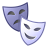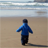# QlikView App Dev

Discussion Board for collaboration related to QlikView App Development.

Announcements
Customer & Partners, DEC. 9, 11 AM ET: Qlik Product & Strategy Roadmap Session: Data Analytics REGISTER NOW
cancel
Showing results for
Did you mean:Contributor III

## calculation based on variable for pivot

Hi Community,

I have a requirement to get the calculation of a expression based on min and max date variable excluding date selection. please help.

Regards.

2 Solutions

Accepted SolutionsChampion

try like this?

=count(<DateField={"\$(= '>=' & date(startdate Variable) & '<=' & date(enddate Variable))"}>} distinct customer)Contributor III
Author

HI Sunny AND Balraj,

Thanks for the reply this is done.i have used this for min date : sum({\$<[DATE Field] = {"<=\$(=vmin)"}>} Inventory)

and for max date:  sum({\$<[DATE Field] = {">=\$(=vmax)"}>} Inventory)

Regards,

Himanshi

3 RepliesMVP

May be something like this:

=Sum({<Date = {"\$(='>=' & Date(vStart) & '<=' & Date(vEnd))"}>}Sales)Champion

try like this?

=count(<DateField={"\$(= '>=' & date(startdate Variable) & '<=' & date(enddate Variable))"}>} distinct customer)Contributor III
Author

HI Sunny AND Balraj,

Thanks for the reply this is done.i have used this for min date : sum({\$<[DATE Field] = {"<=\$(=vmin)"}>} Inventory)

and for max date:  sum({\$<[DATE Field] = {">=\$(=vmax)"}>} Inventory)

Regards,

HimanshiCommunity Browser